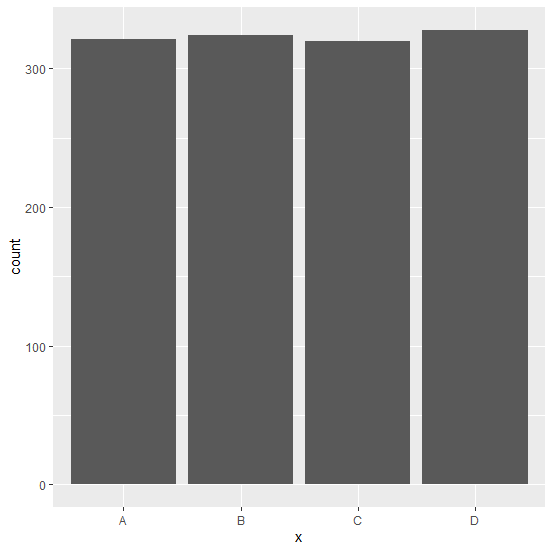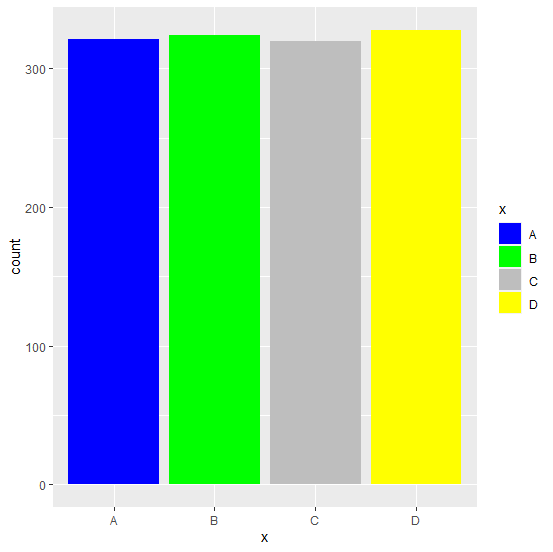# Why scale_fill_manual does not fill the bar with colors created by using ggplot2 in R?

We can manually fill the color of bars in a bar plot which is created by using ggplot2 but for this we would be needing color aesthetics already used for the bars. That means we need to use filling of bars inside aes of ggplot2 and then the colors can be mentioned inside the scale_fill_manual function.

## Example

Consider the below data frame:

Live Demo

> x<-c("A","B","C","D")
> count<-c(321,324,320,328)
> df<-data.frame(x,count)
> df

## Output

x count
1 A 321
2 B 324
3 C 320
4 D 328

## Example

> library(ggplot2)
> ggplot(df,aes(x,count))+geom_bar(stat="identity")

## Output:Creating the bar plot by using scale_fill_manual:

## Example

> ggplot(df,aes(x,count))+geom_bar(stat="identity")+scale_fill_manual(values=c("blue","green","grey","yellow"))

## OutputThis does not change the color of bars.

Creating the bar plot by using scale_fill_manual but passing the fill of bars in aes:

## Example

> ggplot(df,aes(x,count,fill=x))+geom_bar(stat="identity")+scale_fill_manual(values=c("blue","green","grey","yellow"))

## Output: